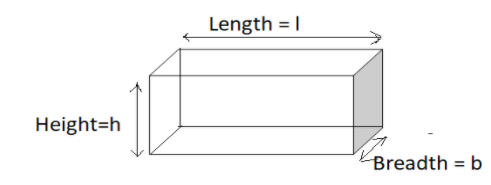QuestionAnswers

# Fill in the blanks:A brick has __________ faces and _______ edges.Verified
59.4k+ views
Hint: A brick is a 3-dimensional solid cuboid. Hence it has 6 rectangles joined together. The face of an object is a flat surface which makes up the object, while an edge is the line on the boundary that joins two vertices together. Now, for a cuboid we have 6 faces which are meeting on 12 lines, so there are 12 edges.Now as we can see, from the figure, the cuboid can be understood to be made up of 6 rectangles. Two rectangles with dimensions $l \times b$ each form the upper face and lower face of the cuboidal brick.
Next, we have two rectangles of dimensions $h \times b$ which make up the two side faces of the cuboidal brick.
Next, we have two rectangles of dimensions $h \times l$ which make up the front and back face of the cuboidal brick.
Note: A cube and a cuboid are very similar having 6 faces. A cube is made up of 6 squares, hence all dimensions of a cube are equal, such that the volume of a cube of side a' units becomes ${a^3}$ while its total surface area is $6{a^2}$ square units.